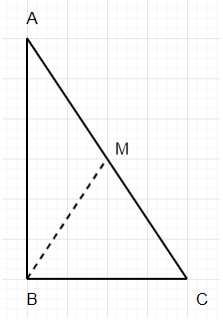# Python program to find angle between mid-point and base of a right angled triangle

PythonServer Side ProgrammingProgramming

#### Beyond Basic Programming - Intermediate Python

Most Popular

36 Lectures 3 hours

#### Practical Machine Learning using Python

Best Seller

91 Lectures 23.5 hours

#### Practical Data Science using Python

22 Lectures 6 hours

Suppose we have two sides of a right angled triangle, these sides are AB and BC. Consider the midpoint of hypotenuse AC is M. We have to find the angle between M and BC.So, if the input is like ab = 6 bc = 4, then the output will be 56.309932474020215 because arc_tan of ab/bc is 0.9828 but in degrees it is 56.31.

To solve this, we will follow these steps −

• ans := arc-tan(ab/bc)
• return ans in degrees

## Example

Let us see the following implementation to get better understanding

from math import atan, pi
def solve(ab, bc):
return 180/pi * rad

ans = deg(atan(ab/bc))
return ans

ab = 6
bc = 4
print(solve(ab, bc))

## Input

6, 4

## Output

45.0